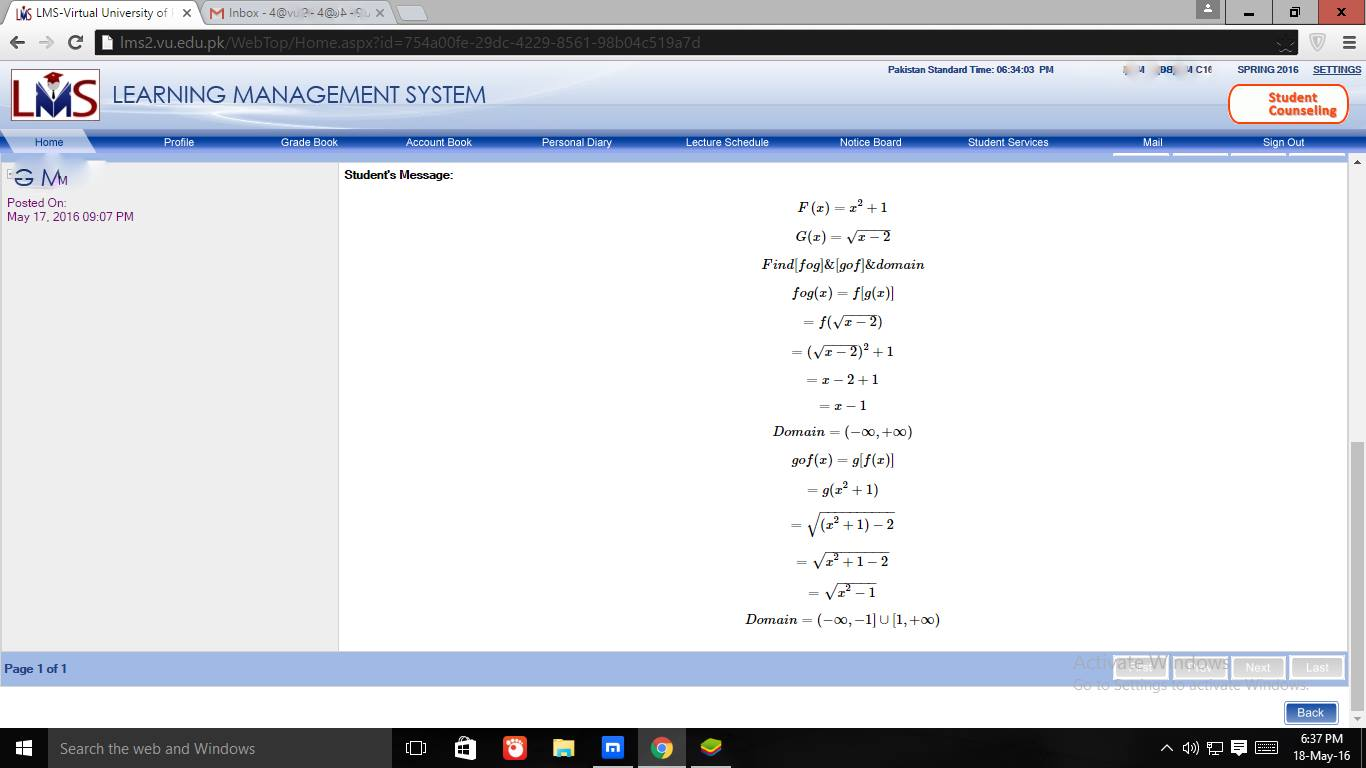We are here with you hands in hands to facilitate your learning & don't appreciate the idea of copying or replicating solutions. Read More>>www.vustudents.ning.com..How to Join Subject Study Groups & Get Helping Material?..Views: 1720

Replies to This Discussion

Our main purpose here discussion not just Solution

We are here with you hands in hands to facilitate your learning and do not appreciate the idea of copying or replicating solutions.

MTH101 - Calculus And Analytical Geometry GDB No. 1 Solution Spring 2016 Due Date May 23, 2016

MTH101 - Calculus And Analytical Geometry GDB No. 1 Solution Spring 2016 Due Date May 23, 2016

For f(x)  = x2+1 and g(x) = x2,find the composition fg and gf and identify the domain of each.Note: Domain of a function means those values of x at which both compositionare not undefined.smjhaaa b dein bhai isay

mth101 gdb solution

Attachments:IDEA SOLUTION ATTACHED

Attachments:Given f(χ) = χ²+1

g(χ) = χ-²

f∘g = (f∘g) (χ) = f(g(χ))

Putting values

=f(√χ-2)

=(√χ-2)²+1

=χ-2+1

=χ-1

Domain= (-∞,∞)

g∘f = (g∘f) (χ)

g = g(f(χ))

= g(χ²+1)

= √(χ²+1) - 2

= √(χ²-1)

Domain = (-∞,-1] , ∪ [1,∞)

thankssss Alot,,,

math type ma square kase lete ha

Correct Solution of mth101 GDB is here
http://machinefreak.com/mth101-gdb-no1-solution